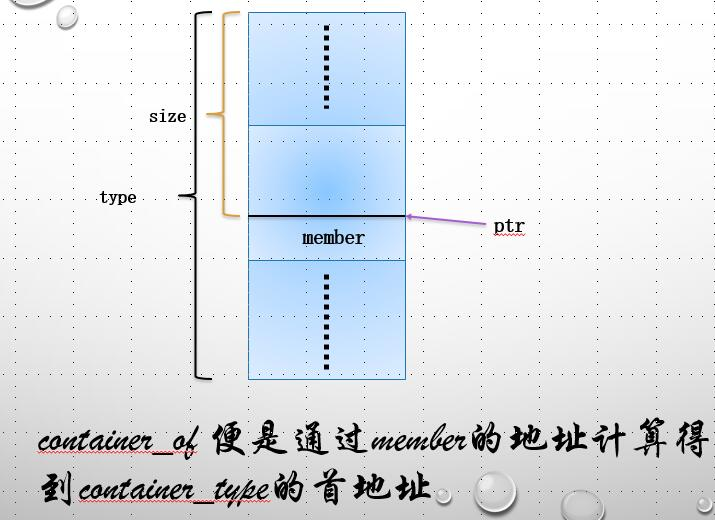## container of()函数与拓展

### container of原理``````              type的起始地址 = ptr - size      (这里需要都转换为char *，因为它为单位字节)。
``````

``````#define container_of(ptr, type, member) ({              \
const typeof( ((type *)0)->member ) *__mptr = (ptr);    \
(type *)( (char *)__mptr - offsetof(type,member) );})
``````

``````#define offsetof(TYPE, MEMBER) ((size_t) &((TYPE *)0)->MEMBER)
``````

### （一）0 指针的使用

``````#include<stdio.h>

struct test
{
char i ;
int j;
char k;
};

int main()
{
struct test temp;
printf("&temp = %p\n",&temp);
printf("&temp.k = %p\n",&temp.k);
printf("&((struct test *)0)->k = %d\n",((int)&((struct test *)0)->k));

}
``````

``````&temp = 0xbf9815b4
&temp.k = 0xbf9815bc
&((struct test *)0)->k = 8
``````

``````#define offsetof(TYPE, MEMBER) ((size_t) &((TYPE *)0)->MEMBER)
``````

### （二） 内核编程的严谨性

``````#define container_of(ptr, type, member) ({              \
const typeof( ((type *)0)->member ) *__mptr = (ptr);    \
(type *)( (char *)__mptr - offsetof(type,member) );})
``````

``````const typeof( ((type *)0)->member ) *__mptr = (ptr);
``````
##### 它的作用是什么呢？

``````typeof( ((type *)0)->member )
``````

### （三） 总结

`container_of（ptr, type,member）`函数的实现包括两部分：

``````       1.  判断ptr 与 member 是否为同意类型

2.  计算size大小，结构体的起始地址 = (type *)((char *)ptr - size)   (注：强转为该结构体指针)
``````

`container_of(ptr,type,member)`,这里面有ptr,type,member分别代表指针、类型、成员。

——————————————————————————————————————————————————————————————

## 测试 和 拓展

``````#include <stdio.h>
#include <stdlib.h>

/* 结构变量MEMBER到结构体首地址的偏移地址 */
#define OFFSET(TYPE,MEMBER) (char)&((TYPE*)0)->MEMBER

/* 通过结构体变量的首地址BODY找到它其中的某个成员BODY->MEMBER的地址
BODY，TYPE_BODY：分别是结构体变量的地址和类型
MEMBER,TYPE_MEMBER：分别是结构体成员的变量名和类型
*/
#define BODY_TO_MEMBER(BODY,TYPE_BODY,MEMBER,TYPE_MEMBER) (TYPE_MEMBER *)((char*)BODY + OFFSET(TYPE_BODY,MEMBER))

/*
通过一个结构变量中一个成员的地址找到这个结构体变量的首地址。
ptr,type,member分别代表指针、类型、成员
*/
#define container_of(ptr,type,member) (type *)((char*)ptr - OFFSET(type,member))

/* 举例测试 */
struct student{
char name;
int num;
};
int main()
{
struct student a;
a.name = '1';
a.num = 10;

printf("%d\n",OFFSET(struct student,num));
printf("%p\n",&a);
printf("%p\n",container_of(&a.num, struct student, num));

printf("%p\n",&a.num);
printf("%p\n",BODY_TO_MEMBER(&a, struct student, num, int));

return 0;
}
``````

0条评论